# How to Calculate and Solve for Horizontal Deflection |Irrigation Water Requirement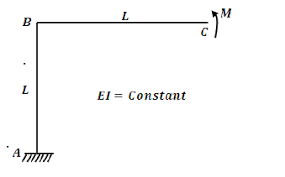The image above represents horizontal deflection.

To compute for horizontal deflection, seven essential parameters are needed and these parameters are Deflection Lag Factor (Dt), Bedding Angle Factor (K), Soil Load per Unit Length (W), Modulus of Elasticity of Tubing (E), Moment of Inertia of Tubing (I), Mean Radius of Tubing (r) and Modulus of Soil Reaction (E’).

The formula for calculating horizontal deflection:

Δx = DtKW / [EI/r³] + 0.061E’

Where:

Δx = Horizontal Deflection
Dt = Deflection Lag Factor
K = Bedding Angle Factor
W = Soil Load per Unit Length
E = Modulus of Elasticity of Tubing
I = Moment of Inertia of Tubing
r = Mean Radius of Tubing
E’ = Modulus of Soil Reaction

Let’s solve an example;
Find the horizontal deflection when the deflection lag factor is 6, the bedding angle factor is 4, the soil load per unit length is 9, the modulus of elasticity of tubing is 10, the moment of inertia of tubing is 2, the mean radius of tubing is 11 and the modulus of soil reaction is 8.

This implies that;

Dt = Deflection Lag Factor = 6
K = Bedding Angle Factor = 4
W = Soil Load per Unit Length = 9
E = Modulus of Elasticity of Tubing = 10
I = Moment of Inertia of Tubing = 2
r = Mean Radius of Tubing = 11
E’ = Modulus of Soil Reaction = 8

Δx = DtKW / [EI/r³] + 0.061E’
Δx = (6)(4)(9) / [(10)(2)/(11)³] + 0.061(8)
Δx = 216 / [20/1331] + 0.488
Δx = 216 / [0.015] + 0.488
Δx = 216 / 0.50
Δx = 429.40

Therefore, the horizontal deflection is 429.40.

Nickzom Calculator – The Calculator Encyclopedia is capable of calculating the horizontal deflection.

To get the answer and workings of the horizontal deflection using the Nickzom Calculator – The Calculator Encyclopedia. First, you need to obtain the app.

You can get this app via any of these means:

To get access to the professional version via web, you need to register and subscribe for NGN 2,000 per annum to have utter access to all functionalities.
You can also try the demo version via https://www.nickzom.org/calculator

Apple (Paid) – https://itunes.apple.com/us/app/nickzom-calculator/id1331162702?mt=8
Once, you have obtained the calculator encyclopedia app, proceed to the Calculator Map, then click on Agricultural under Engineering.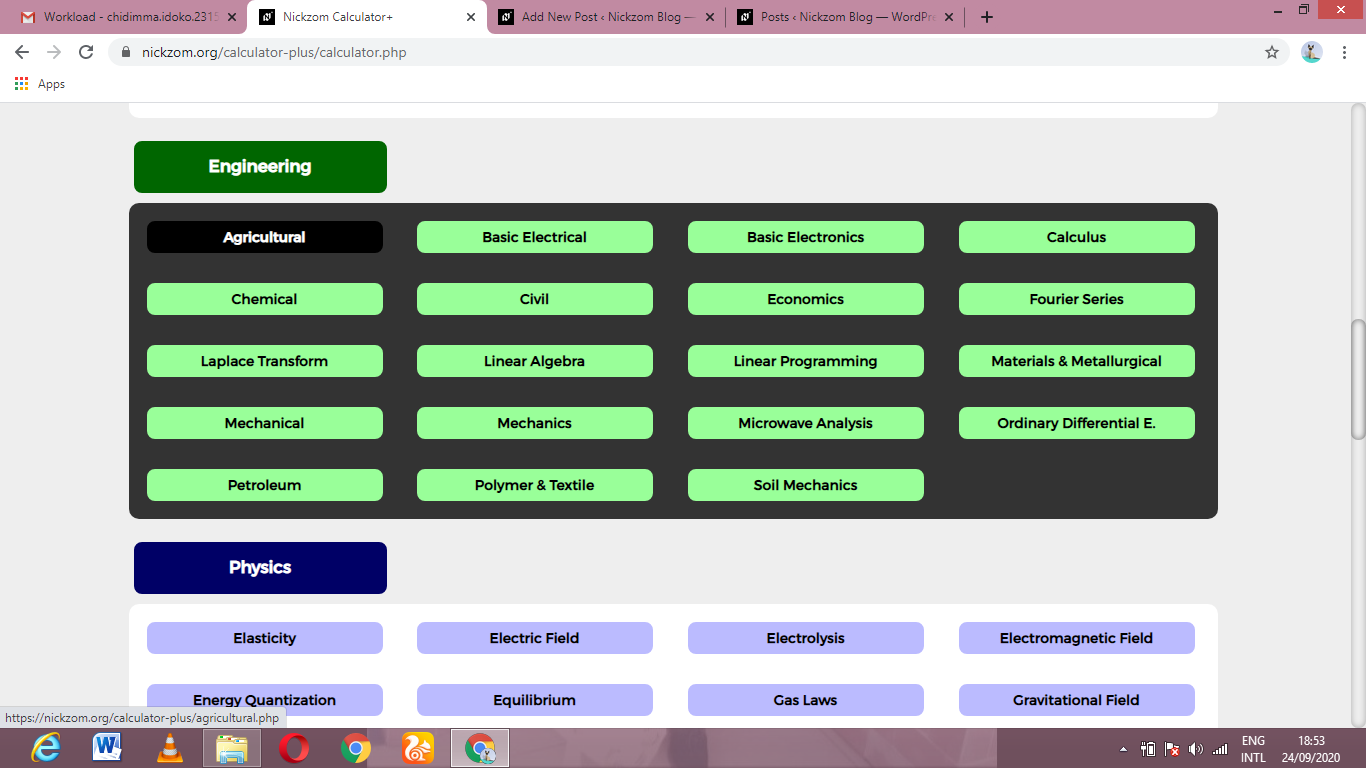Now, Click on Irrigation Water Requirement under Agricultural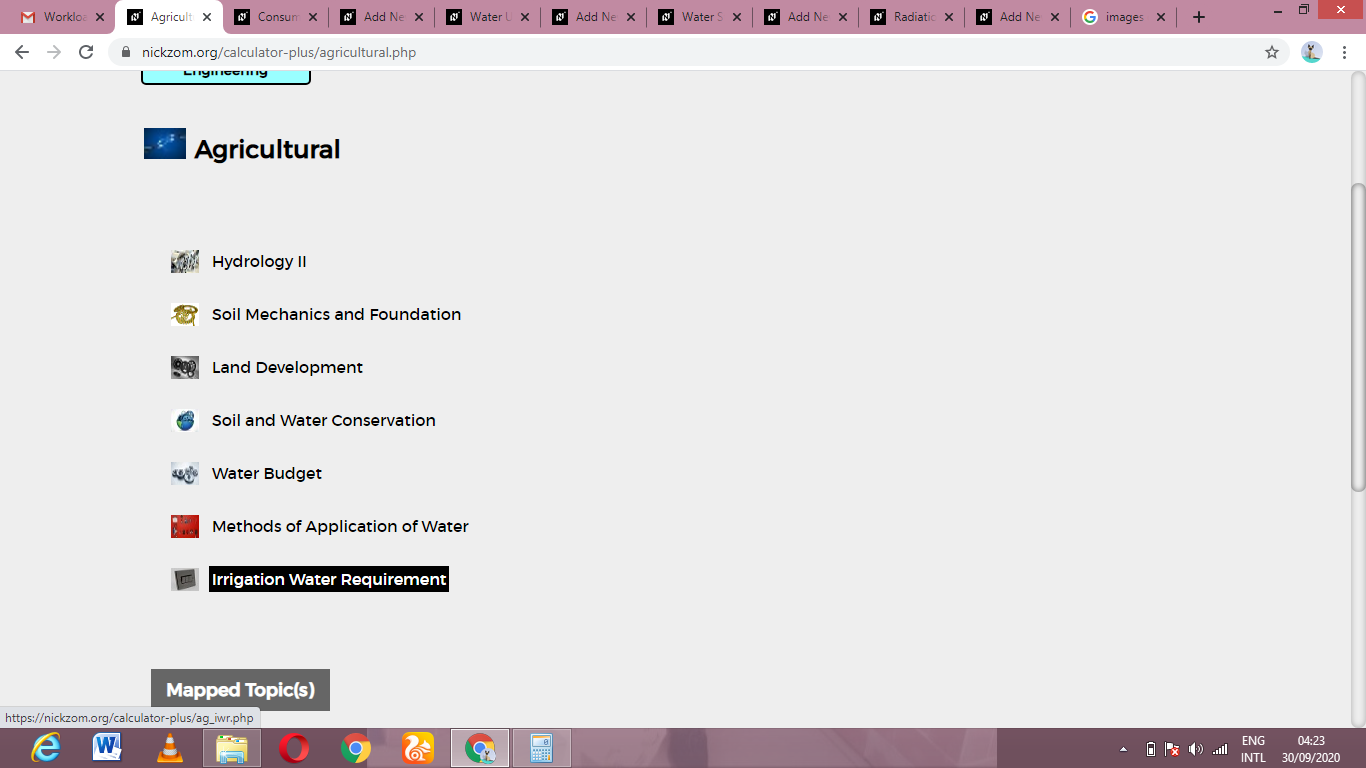Now, Click on Horizontal Deflection under Irrigation Water Requirement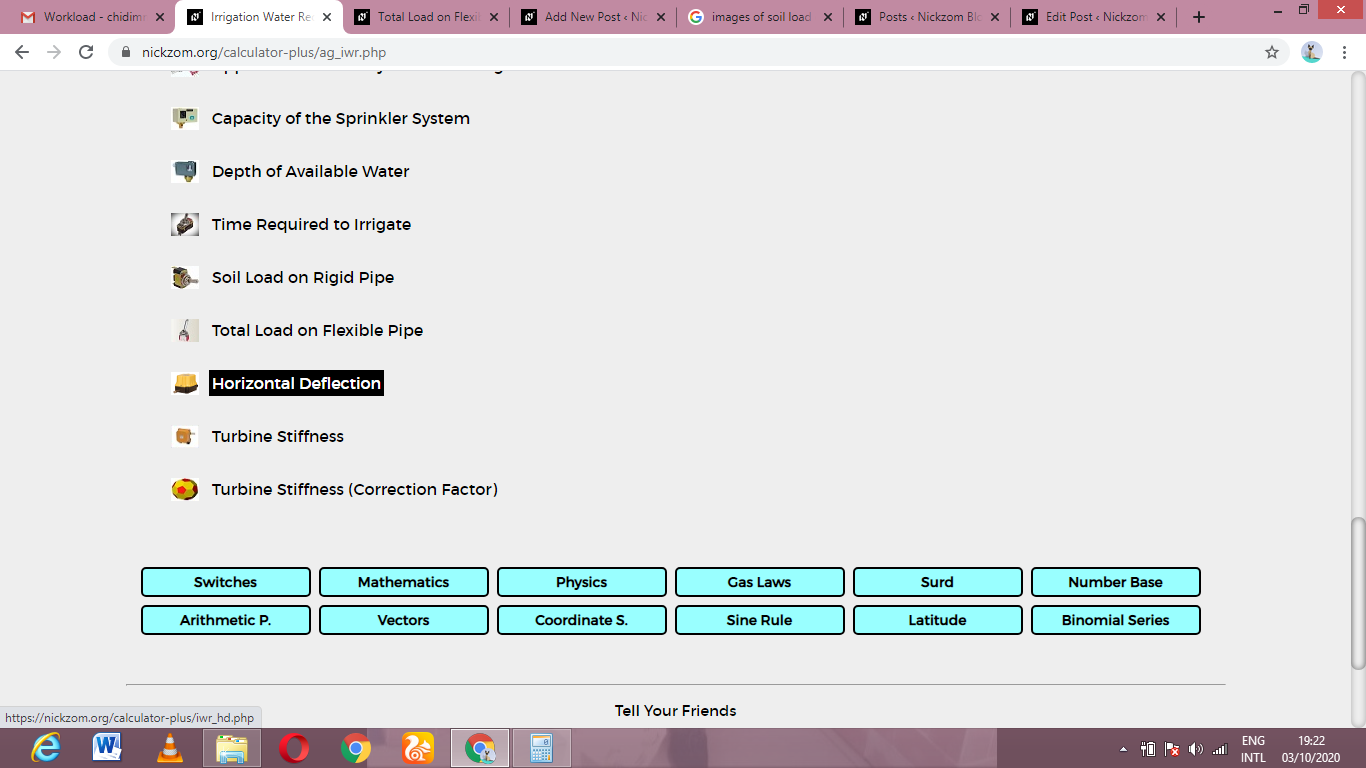The screenshot below displays the page or activity to enter your values, to get the answer for the horizontal deflection according to the respective parameters which is the Deflection Lag Factor (Dt), Bedding Angle Factor (K), Soil Load per Unit Length (W), Modulus of Elasticity of Tubing (E), Moment of Inertia of Tubing (I), Mean Radius of Tubing (r) and Modulus of Soil Reaction (E’).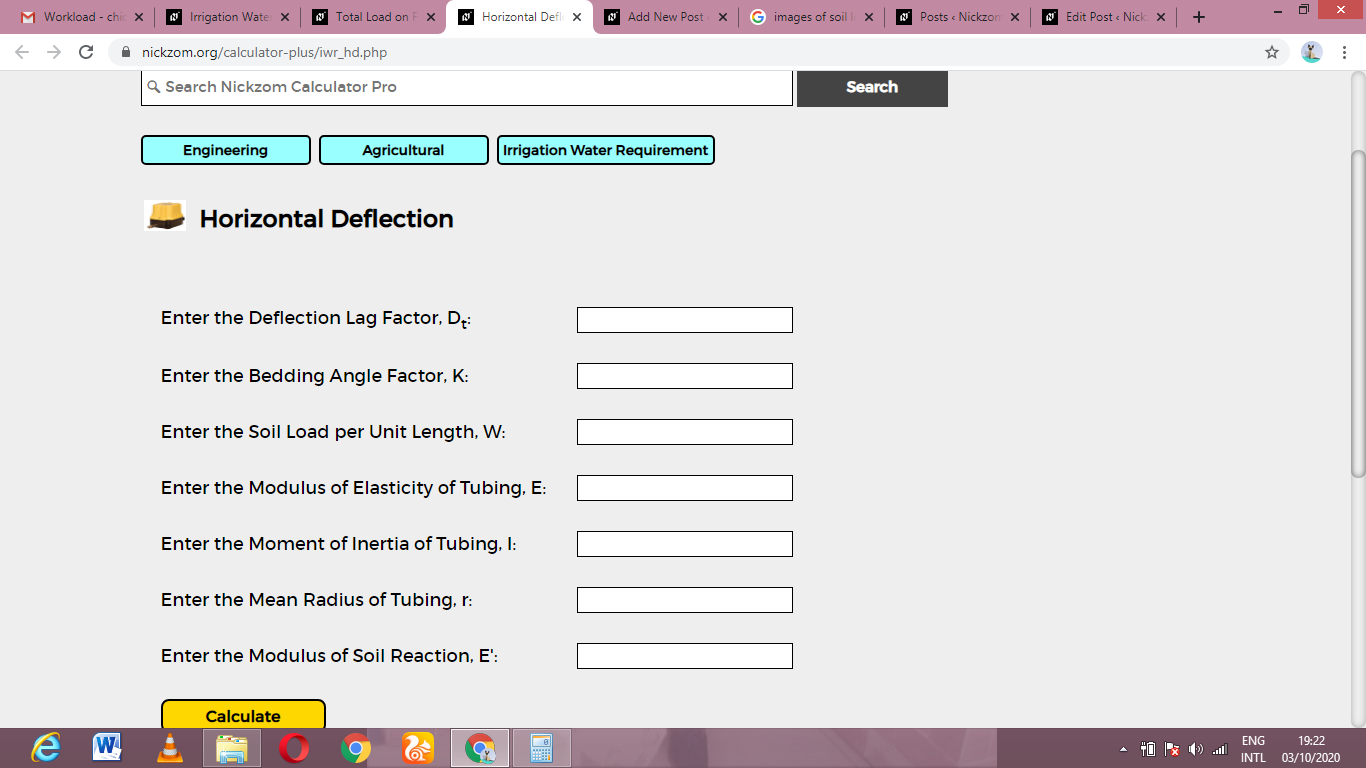Now, enter the values appropriately and accordingly for the parameters as required by the Deflection Lag Factor (Dt) is 6, Bedding Angle Factor (K) is 4, Soil Load per Unit Length (W) is 9, Modulus of Elasticity of Tubing (E) is 10, Moment of Inertia of Tubing (I) is 2, Mean Radius of Tubing (r) is 11 and Modulus of Soil Reaction (E’) is 8.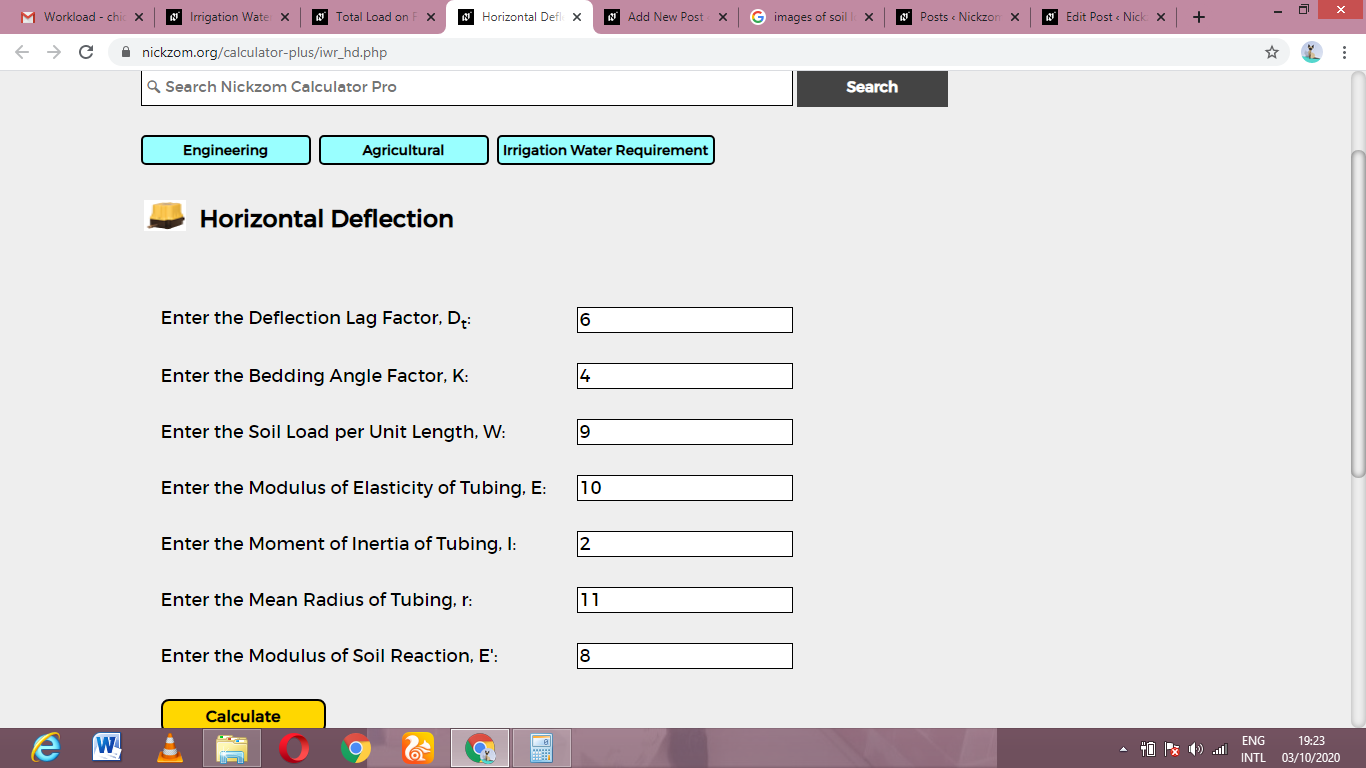Finally, Click on Calculate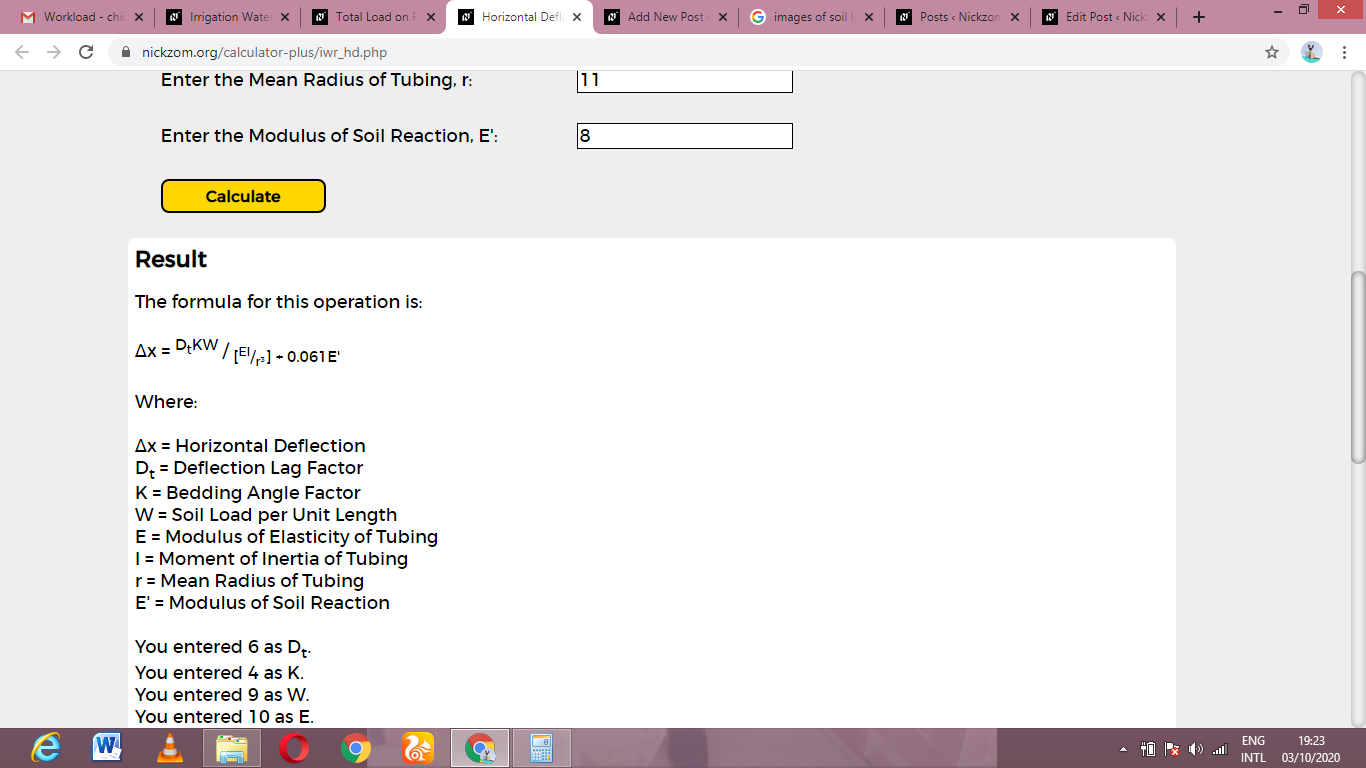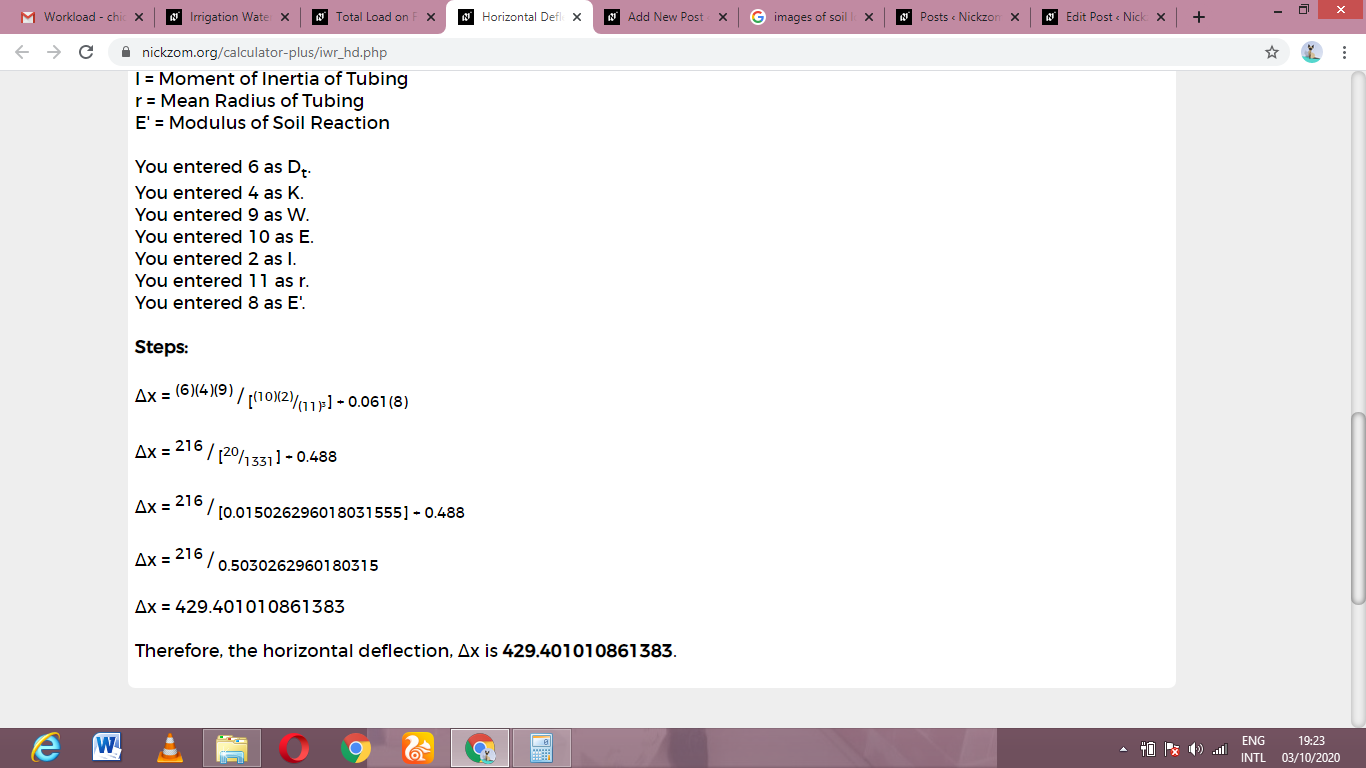As you can see from the screenshot above, Nickzom Calculator– The Calculator Encyclopedia solves for the horizontal deflection and presents the formula, workings and steps too.